Скачать презентацию Part 4 Fluids Heat First section Pressure

8c27af84b742173c6b3fcbecd22a136e.ppt

• Количество слайдов: 49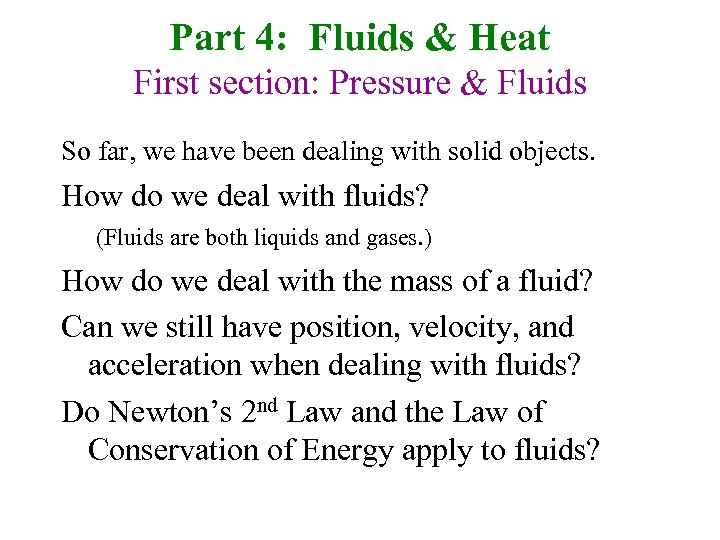Part 4: Fluids & Heat First section: Pressure & Fluids So far, we have been dealing with solid objects. How do we deal with fluids? (Fluids are both liquids and gases. ) How do we deal with the mass of a fluid? Can we still have position, velocity, and acceleration when dealing with fluids? Do Newton’s 2 nd Law and the Law of Conservation of Energy apply to fluids?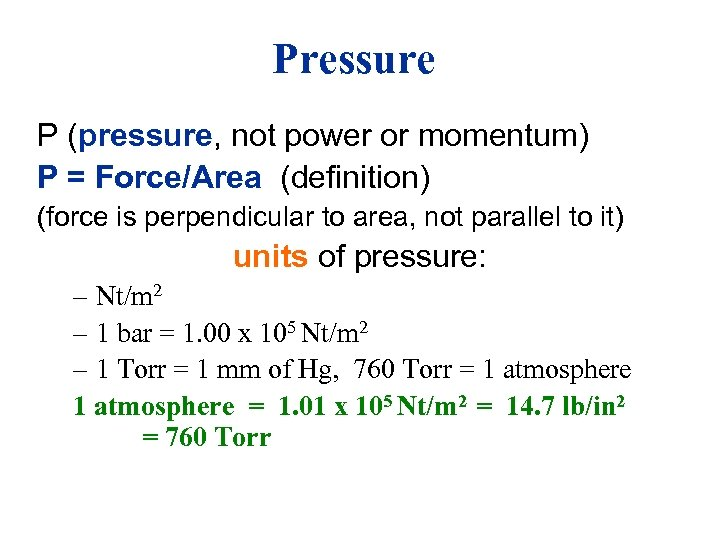Pressure P (pressure, not power or momentum) P = Force/Area (definition) (force is perpendicular to area, not parallel to it) units of pressure: – Nt/m 2 – 1 bar = 1. 00 x 105 Nt/m 2 – 1 Torr = 1 mm of Hg, 760 Torr = 1 atmosphere = 1. 01 x 105 Nt/m 2 = 14. 7 lb/in 2 = 760 Torr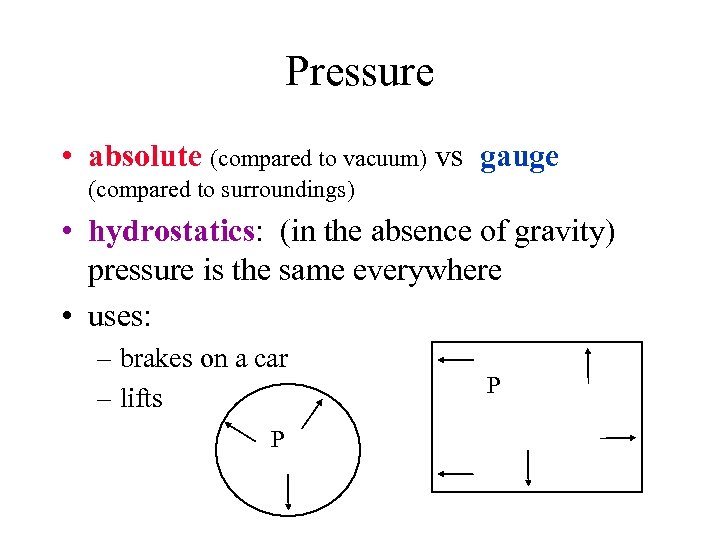Pressure • absolute (compared to vacuum) vs gauge (compared to surroundings) • hydrostatics: (in the absence of gravity) pressure is the same everywhere • uses: – brakes on a car – lifts P P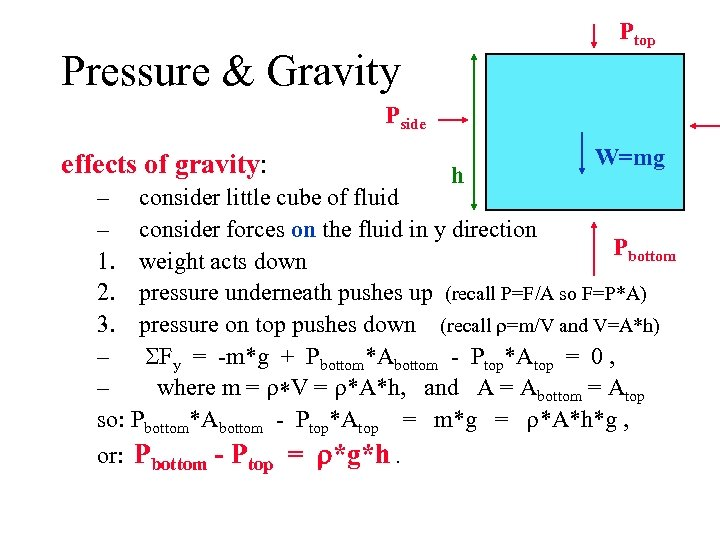Ptop Pressure & Gravity Pside effects of gravity: h W=mg – consider little cube of fluid – consider forces on the fluid in y direction Pbottom 1. weight acts down 2. pressure underneath pushes up (recall P=F/A so F=P*A) 3. pressure on top pushes down (recall =m/V and V=A*h) – Fy = -m*g + Pbottom*Abottom - Ptop*Atop = 0 , – where m = V = *A*h, and A = Abottom = Atop so: Pbottom*Abottom - Ptop*Atop = m*g = *A*h*g , or: Pbottom - Ptop = *g*h.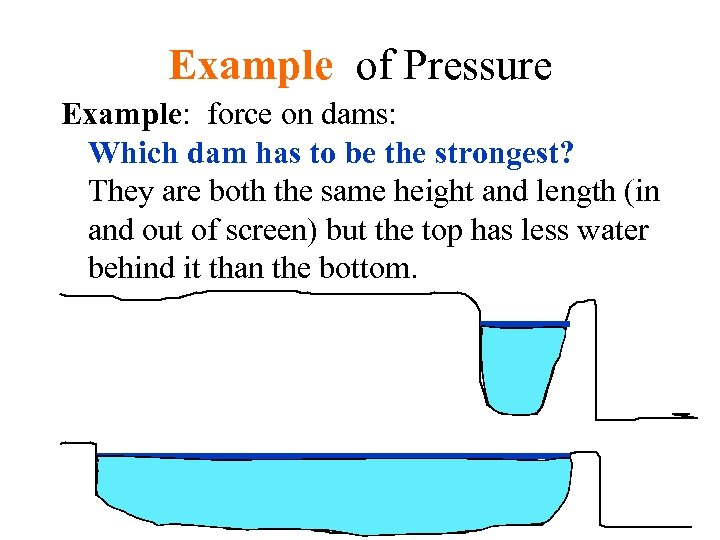Example of Pressure Example: force on dams: Which dam has to be the strongest? They are both the same height and length (in and out of screen) but the top has less water behind it than the bottom.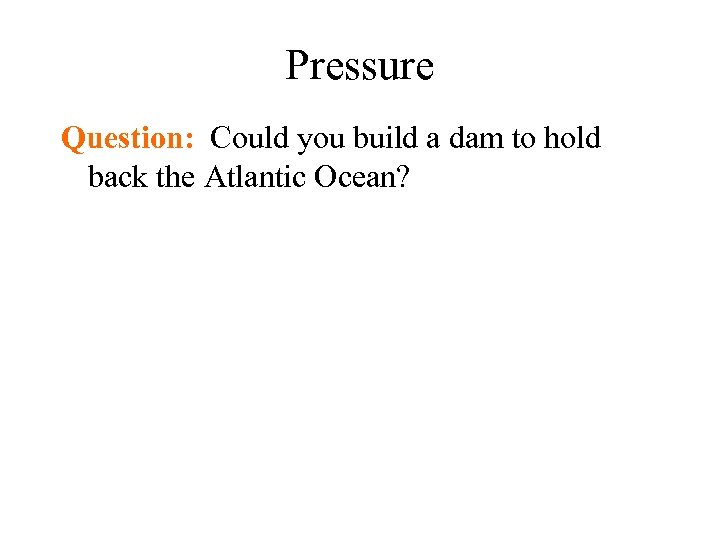Pressure Question: Could you build a dam to hold back the Atlantic Ocean?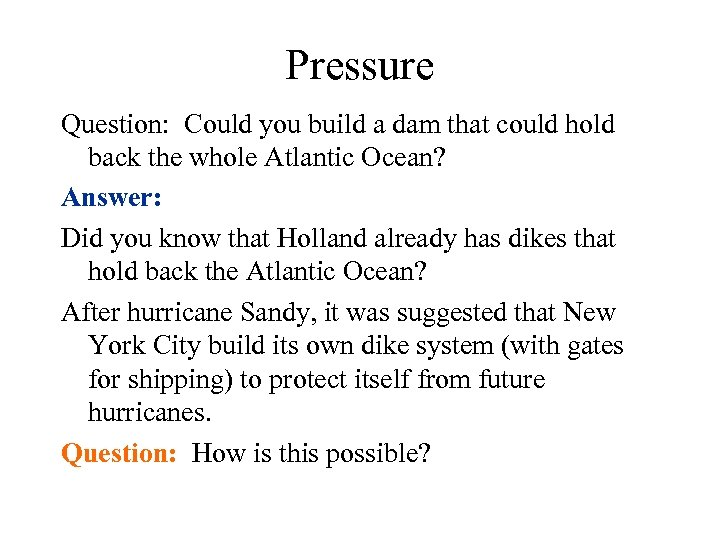Pressure Question: Could you build a dam that could hold back the whole Atlantic Ocean? Answer: Did you know that Holland already has dikes that hold back the Atlantic Ocean? After hurricane Sandy, it was suggested that New York City build its own dike system (with gates for shipping) to protect itself from future hurricanes. Question: How is this possible?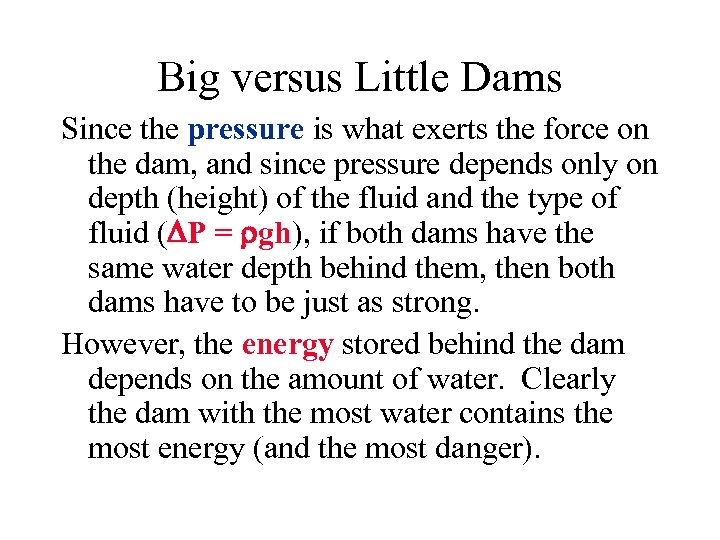Big versus Little Dams Since the pressure is what exerts the force on the dam, and since pressure depends only on depth (height) of the fluid and the type of fluid ( P = gh), if both dams have the same water depth behind them, then both dams have to be just as strong. However, the energy stored behind the dam depends on the amount of water. Clearly the dam with the most water contains the most energy (and the most danger).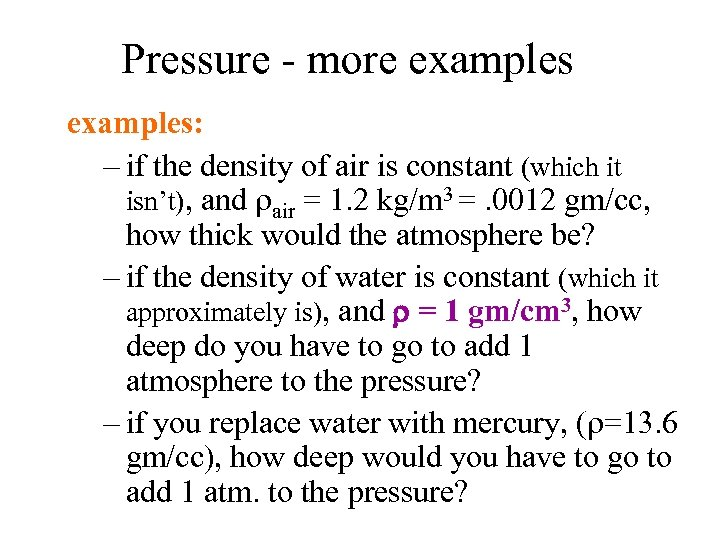Pressure - more examples: – if the density of air is constant (which it isn’t), and air = 1. 2 kg/m 3 =. 0012 gm/cc, how thick would the atmosphere be? – if the density of water is constant (which it approximately is), and = 1 gm/cm 3, how deep do you have to go to add 1 atmosphere to the pressure? – if you replace water with mercury, ( =13. 6 gm/cc), how deep would you have to go to add 1 atm. to the pressure?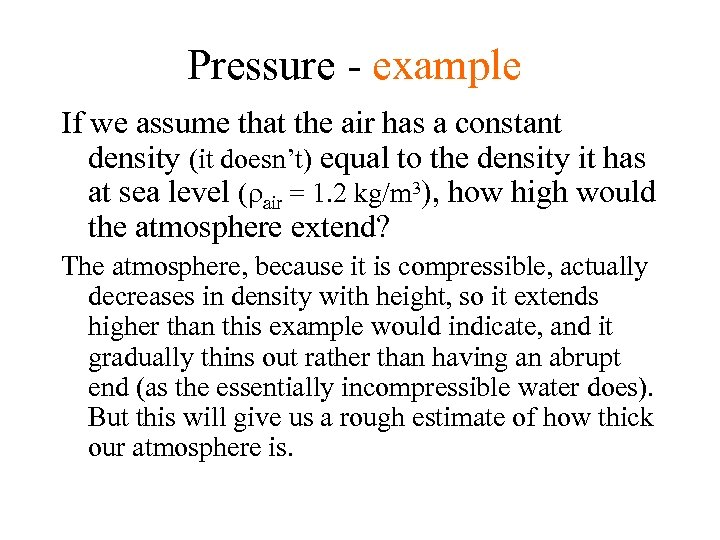Pressure - example If we assume that the air has a constant density (it doesn’t) equal to the density it has at sea level ( air = 1. 2 kg/m 3), how high would the atmosphere extend? The atmosphere, because it is compressible, actually decreases in density with height, so it extends higher than this example would indicate, and it gradually thins out rather than having an abrupt end (as the essentially incompressible water does). But this will give us a rough estimate of how thick our atmosphere is.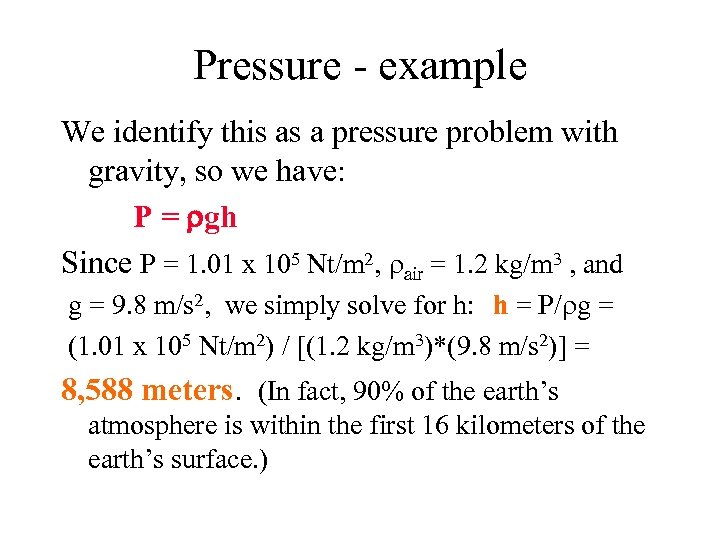Pressure - example We identify this as a pressure problem with gravity, so we have: P = gh Since P = 1. 01 x 105 Nt/m 2, air = 1. 2 kg/m 3 , and g = 9. 8 m/s 2, we simply solve for h: h = P/ g = (1. 01 x 105 Nt/m 2) / [(1. 2 kg/m 3)*(9. 8 m/s 2)] = 8, 588 meters. (In fact, 90% of the earth’s atmosphere is within the first 16 kilometers of the earth’s surface. )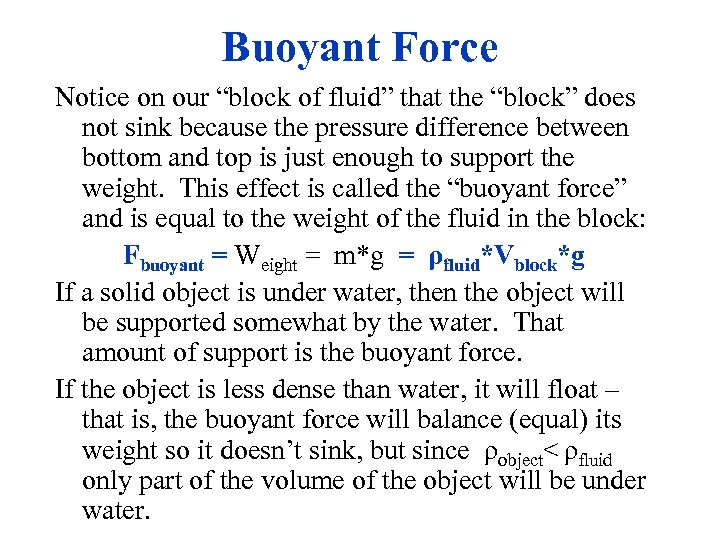Buoyant Force Notice on our “block of fluid” that the “block” does not sink because the pressure difference between bottom and top is just enough to support the weight. This effect is called the “buoyant force” and is equal to the weight of the fluid in the block: Fbuoyant = Weight = m*g = ρfluid*Vblock*g If a solid object is under water, then the object will be supported somewhat by the water. That amount of support is the buoyant force. If the object is less dense than water, it will float – that is, the buoyant force will balance (equal) its weight so it doesn’t sink, but since ρobject< ρfluid only part of the volume of the object will be under water.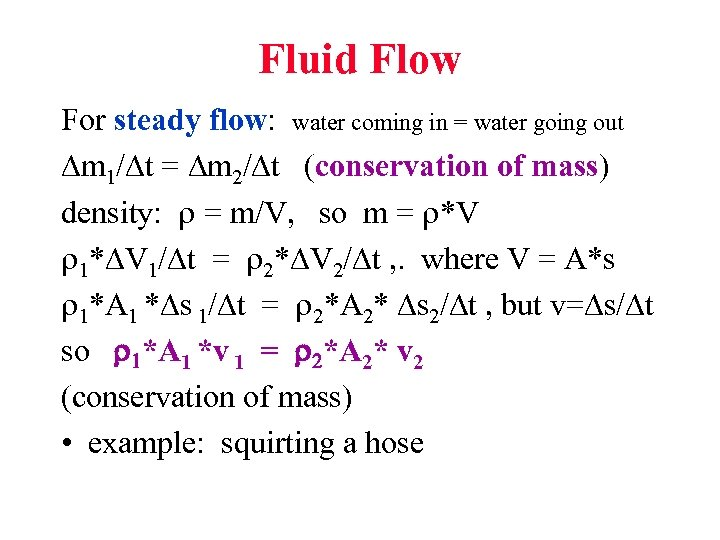Fluid Flow For steady flow: water coming in = water going out m 1/ t = m 2/ t (conservation of mass) density: = m/V, so m = *V * V 1/ t = * V 2/ t , . where V = A*s *A 1 * s 1/ t = *A 2* s 2/ t , but v= s/ t so *A 1 *v 1 = *A 2* v 2 (conservation of mass) • example: squirting a hose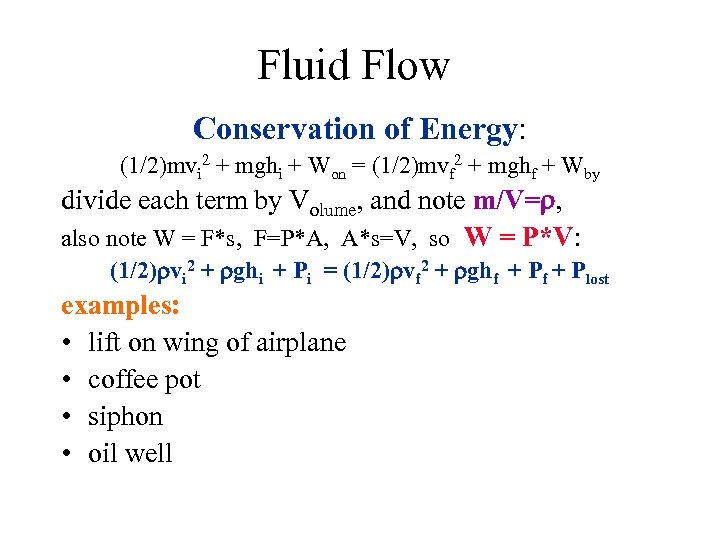Fluid Flow Conservation of Energy: (1/2)mvi 2 + mghi + Won = (1/2)mvf 2 + mghf + Wby divide each term by Volume, and note m/V= , also note W = F*s, F=P*A, A*s=V, so W = P*V: (1/2) vi 2 + ghi + Pi = (1/2) vf 2 + ghf + Plost examples: • lift on wing of airplane • coffee pot • siphon • oil well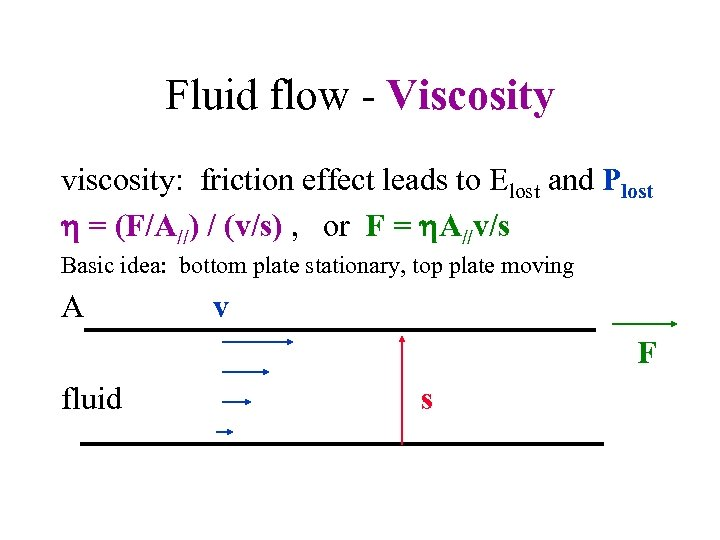Fluid flow - Viscosity viscosity: friction effect leads to Elost and Plost = (F/A//) / (v/s) , or F = A//v/s Basic idea: bottom plate stationary, top plate moving v F fluid s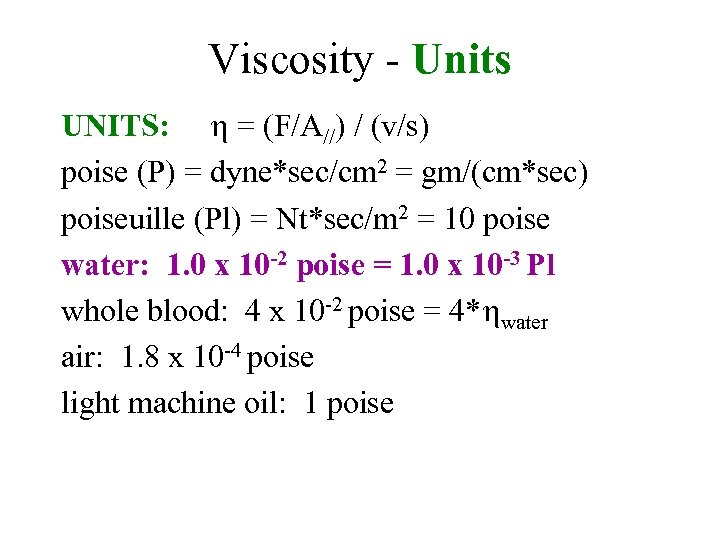Viscosity - Units UNITS: = (F/A//) / (v/s) poise (P) = dyne*sec/cm 2 = gm/(cm*sec) poiseuille (Pl) = Nt*sec/m 2 = 10 poise water: 1. 0 x 10 -2 poise = 1. 0 x 10 -3 Pl whole blood: 4 x 10 -2 poise = 4* water air: 1. 8 x 10 -4 poise light machine oil: 1 poise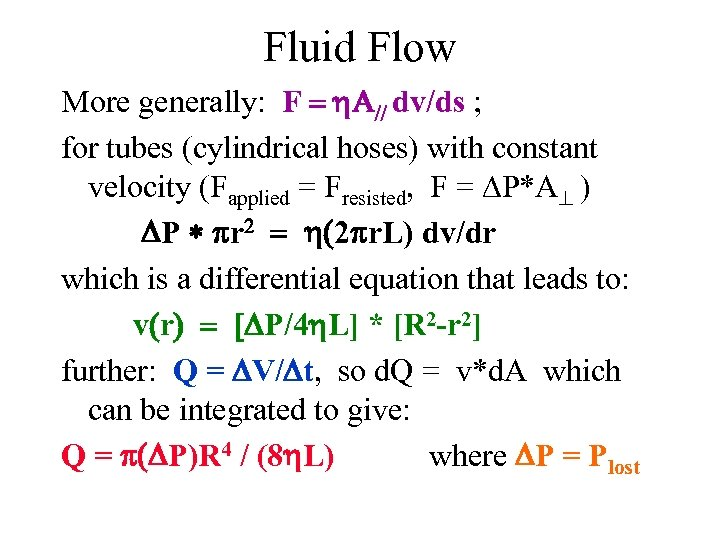Fluid Flow More generally: F dv/ds ; for tubes (cylindrical hoses) with constant velocity (Fapplied = Fresisted, F = P*A ) P r 2 r. L) dv/dr which is a differential equation that leads to: v r P/4 L] * [R 2 -r 2] further: Q = V/ t, so d. Q = v*d. A which can be integrated to give: Q = P)R 4 / (8 L) where P = Plost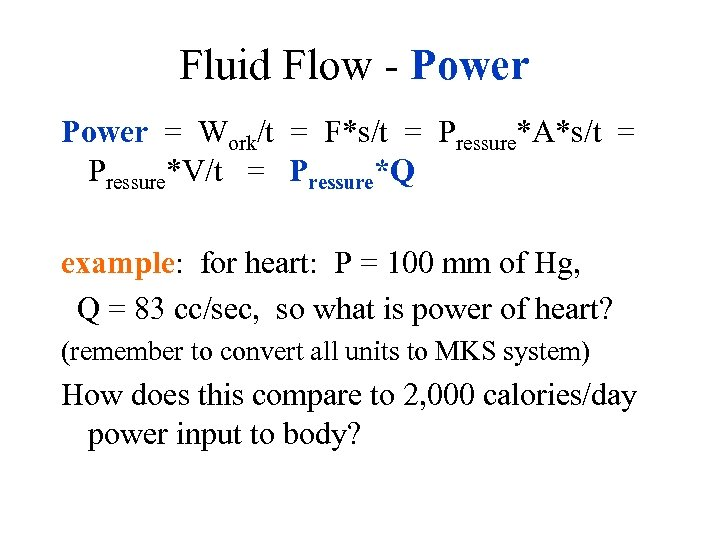Fluid Flow - Power = Work/t = F*s/t = Pressure*A*s/t = Pressure*V/t = Pressure*Q example: for heart: P = 100 mm of Hg, Q = 83 cc/sec, so what is power of heart? (remember to convert all units to MKS system) How does this compare to 2, 000 calories/day power input to body?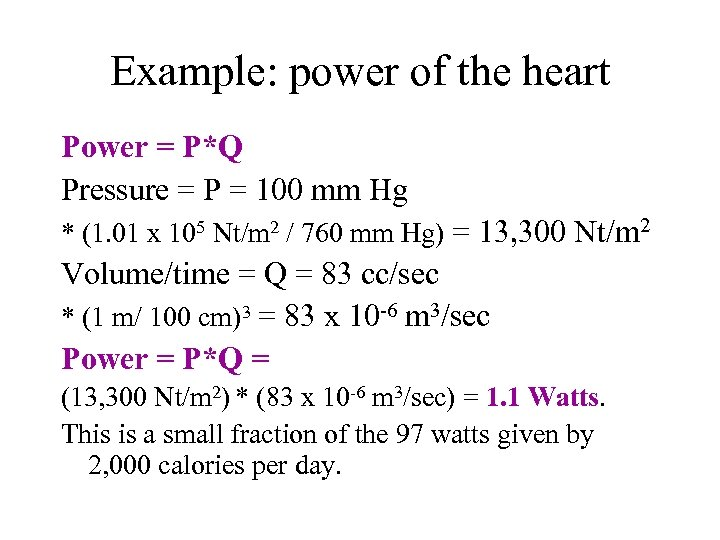Example: power of the heart Power = P*Q Pressure = P = 100 mm Hg * (1. 01 x 105 Nt/m 2 / 760 mm Hg) = 13, 300 Nt/m 2 Volume/time = Q = 83 cc/sec * (1 m/ 100 cm)3 = 83 x 10 -6 m 3/sec Power = P*Q = (13, 300 Nt/m 2) * (83 x 10 -6 m 3/sec) = 1. 1 Watts. This is a small fraction of the 97 watts given by 2, 000 calories per day.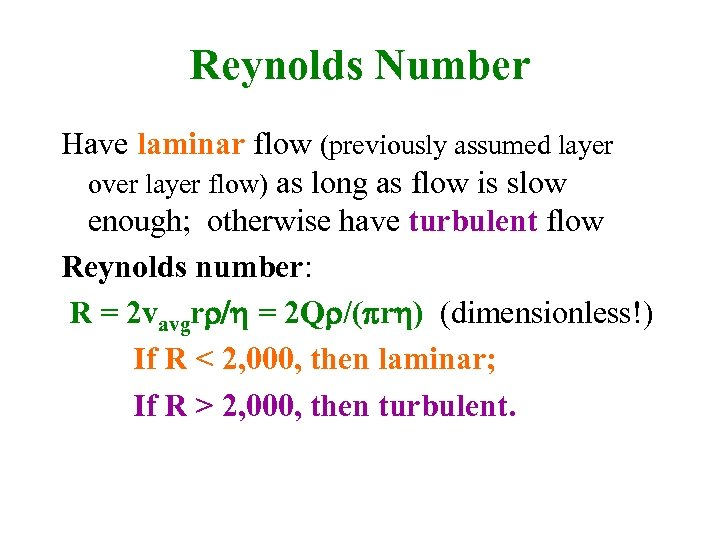Reynolds Number Have laminar flow (previously assumed layer over layer flow) as long as flow is slow enough; otherwise have turbulent flow Reynolds number: R = 2 vavgr = 2 Q /( r ) (dimensionless!) If R < 2, 000, then laminar; If R > 2, 000, then turbulent.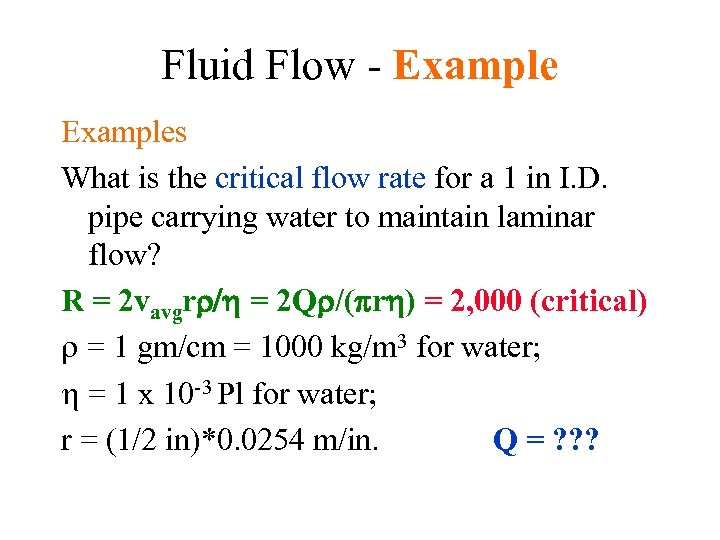Fluid Flow - Examples What is the critical flow rate for a 1 in I. D. pipe carrying water to maintain laminar flow? R = 2 vavgr = 2 Q /( r ) = 2, 000 (critical) = 1 gm/cm = 1000 kg/m 3 for water; = 1 x 10 -3 Pl for water; r = (1/2 in)*0. 0254 m/in. Q = ? ? ?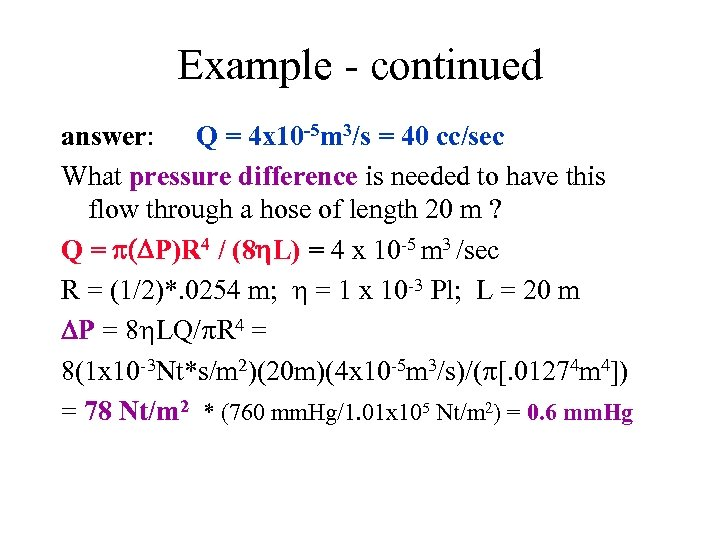Example - continued answer: Q = 4 x 10 -5 m 3/s = 40 cc/sec What pressure difference is needed to have this flow through a hose of length 20 m ? Q = P)R 4 / (8 L) = 4 x 10 -5 m 3 /sec R = (1/2)*. 0254 m; = 1 x 10 -3 Pl; L = 20 m P = 8 LQ/ R 4 = 8(1 x 10 -3 Nt*s/m 2)(20 m)(4 x 10 -5 m 3/s)/( [. 01274 m 4]) = 78 Nt/m 2 * (760 mm. Hg/1. 01 x 105 Nt/m 2) = 0. 6 mm. Hg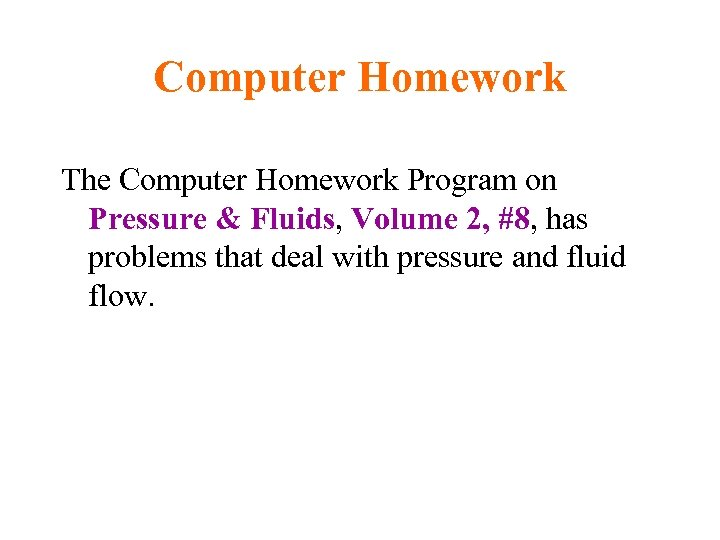Computer Homework The Computer Homework Program on Pressure & Fluids, Volume 2, #8, has problems that deal with pressure and fluid flow.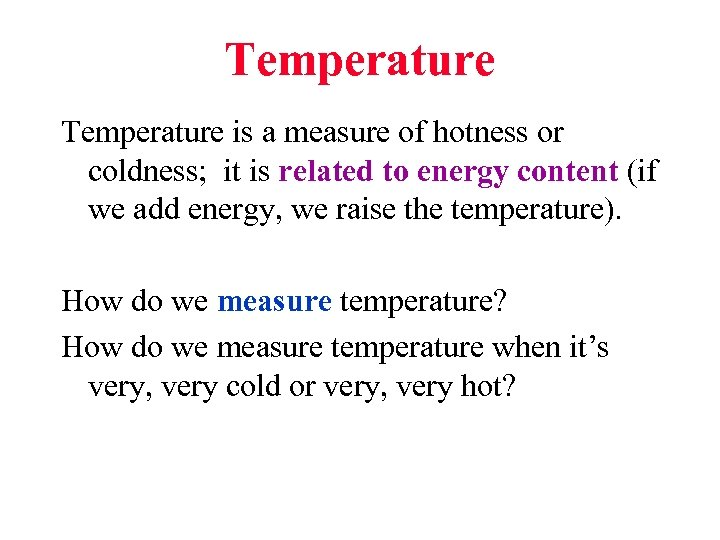Temperature is a measure of hotness or coldness; it is related to energy content (if we add energy, we raise the temperature). How do we measure temperature? How do we measure temperature when it’s very, very cold or very, very hot?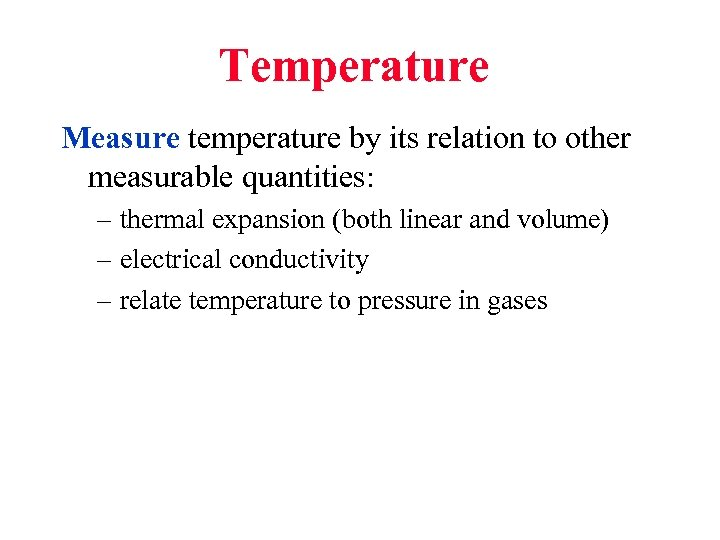Temperature Measure temperature by its relation to other measurable quantities: – thermal expansion (both linear and volume) – electrical conductivity – relate temperature to pressure in gases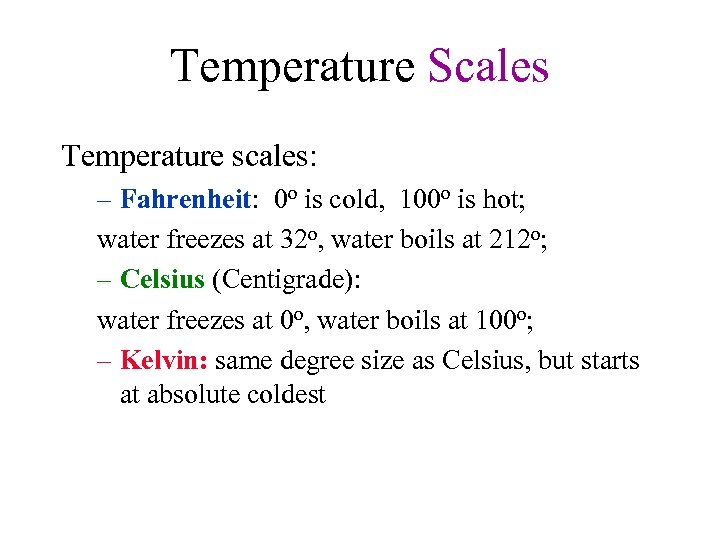Temperature Scales Temperature scales: – Fahrenheit: 0 o is cold, 100 o is hot; water freezes at 32 o, water boils at 212 o; – Celsius (Centigrade): water freezes at 0 o, water boils at 100 o; – Kelvin: same degree size as Celsius, but starts at absolute coldest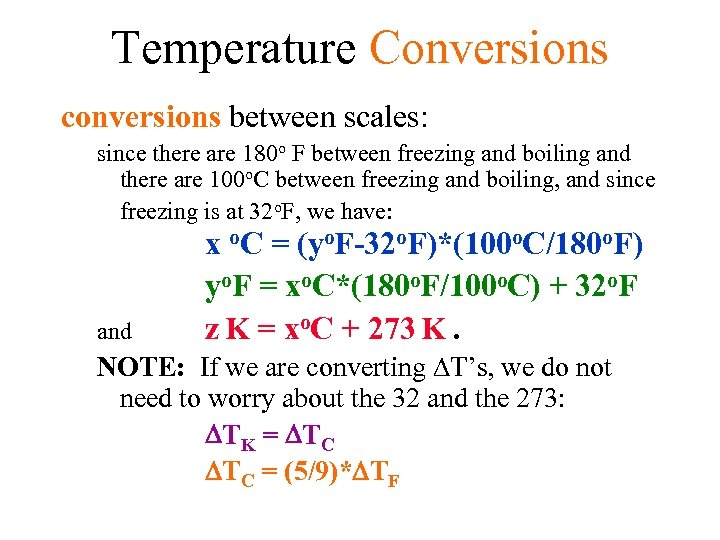Temperature Conversions conversions between scales: since there are 180 o F between freezing and boiling and there are 100 o. C between freezing and boiling, and since freezing is at 32 o. F, we have: and x o. C = (yo. F-32 o. F)*(100 o. C/180 o. F) yo. F = xo. C*(180 o. F/100 o. C) + 32 o. F z K = xo. C + 273 K. NOTE: If we are converting T’s, we do not need to worry about the 32 and the 273: TK = TC TC = (5/9)* TF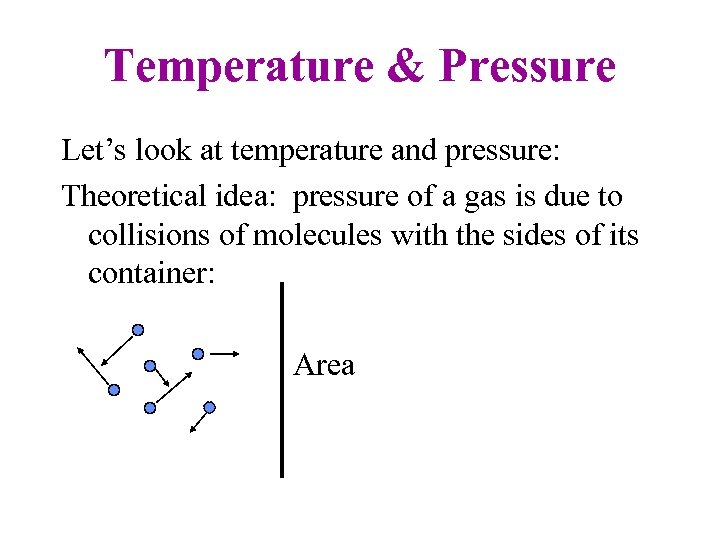Temperature & Pressure Let’s look at temperature and pressure: Theoretical idea: pressure of a gas is due to collisions of molecules with the sides of its container: Area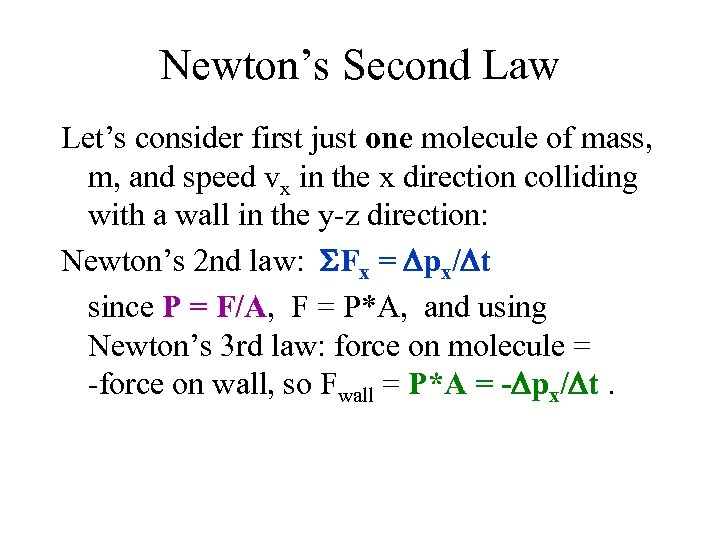Newton’s Second Law Let’s consider first just one molecule of mass, m, and speed vx in the x direction colliding with a wall in the y-z direction: Newton’s 2 nd law: Fx = px/ t since P = F/A, F = P*A, and using Newton’s 3 rd law: force on molecule = -force on wall, so Fwall = P*A = - px/ t.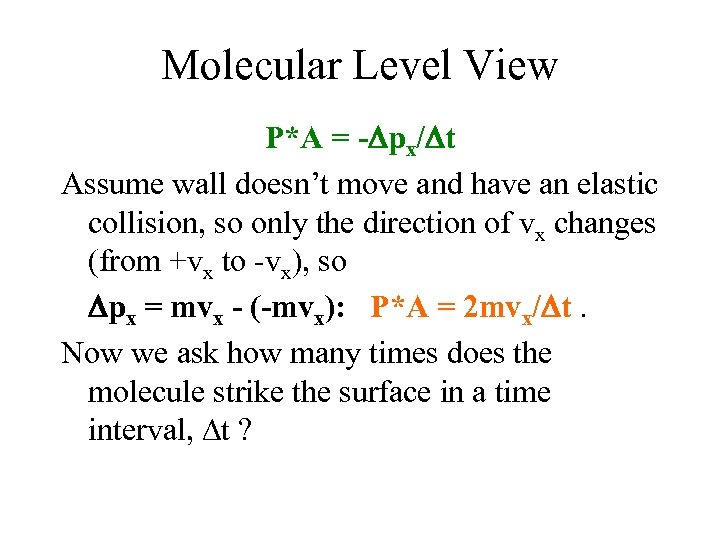Molecular Level View P*A = - px/ t Assume wall doesn’t move and have an elastic collision, so only the direction of vx changes (from +vx to -vx), so px = mvx - (-mvx): P*A = 2 mvx/ t. Now we ask how many times does the molecule strike the surface in a time interval, t ?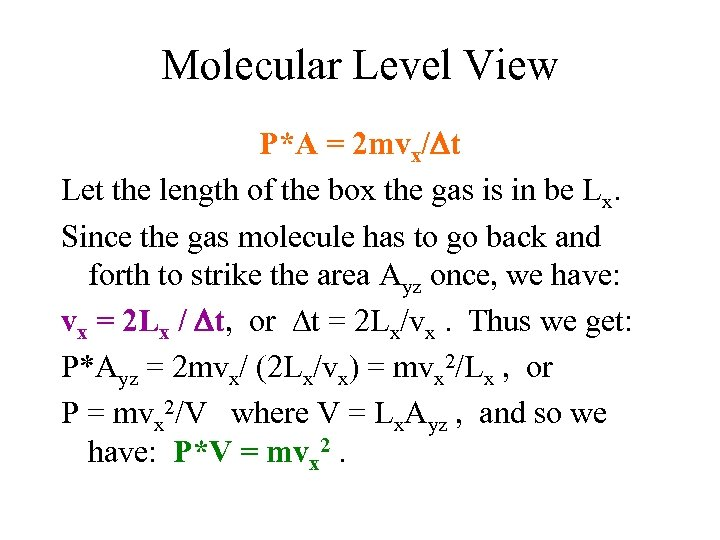Molecular Level View P*A = 2 mvx/ t Let the length of the box the gas is in be Lx. Since the gas molecule has to go back and forth to strike the area Ayz once, we have: vx = 2 Lx / t, or t = 2 Lx/vx. Thus we get: P*Ayz = 2 mvx/ (2 Lx/vx) = mvx 2/Lx , or P = mvx 2/V where V = Lx. Ayz , and so we have: P*V = mvx 2.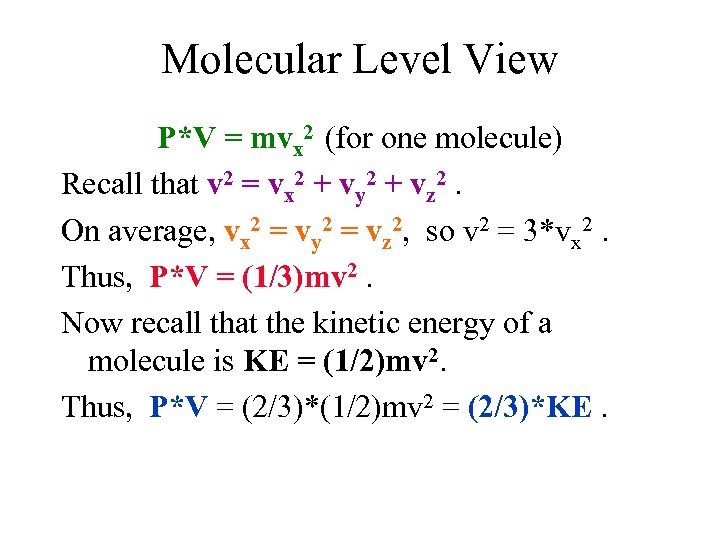Molecular Level View P*V = mvx 2 (for one molecule) Recall that v 2 = vx 2 + vy 2 + vz 2. On average, vx 2 = vy 2 = vz 2, so v 2 = 3*vx 2. Thus, P*V = (1/3)mv 2. Now recall that the kinetic energy of a molecule is KE = (1/2)mv 2. Thus, P*V = (2/3)*(1/2)mv 2 = (2/3)*KE.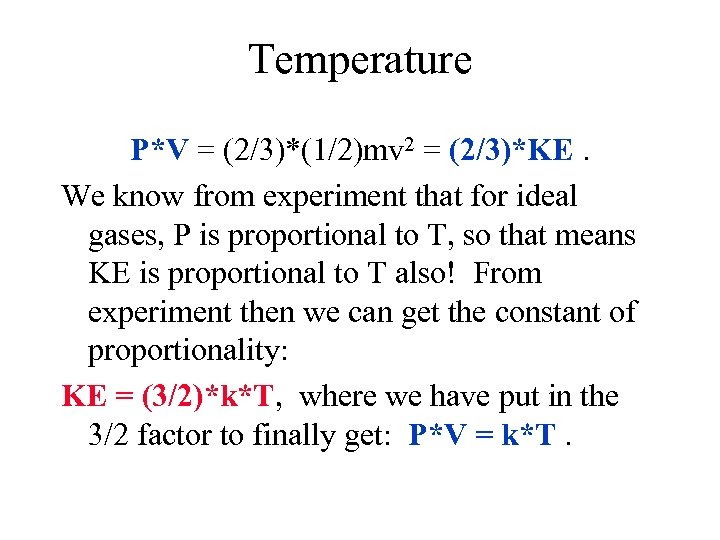Temperature P*V = (2/3)*(1/2)mv 2 = (2/3)*KE. We know from experiment that for ideal gases, P is proportional to T, so that means KE is proportional to T also! From experiment then we can get the constant of proportionality: KE = (3/2)*k*T, where we have put in the 3/2 factor to finally get: P*V = k*T.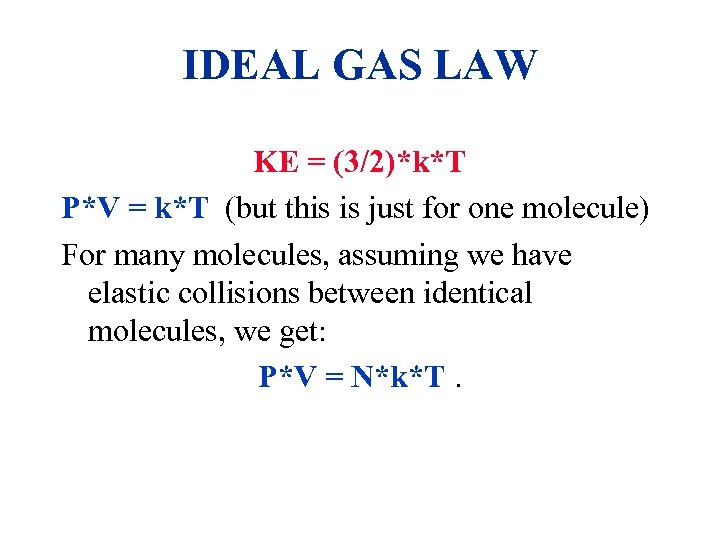IDEAL GAS LAW KE = (3/2)*k*T P*V = k*T (but this is just for one molecule) For many molecules, assuming we have elastic collisions between identical molecules, we get: P*V = N*k*T.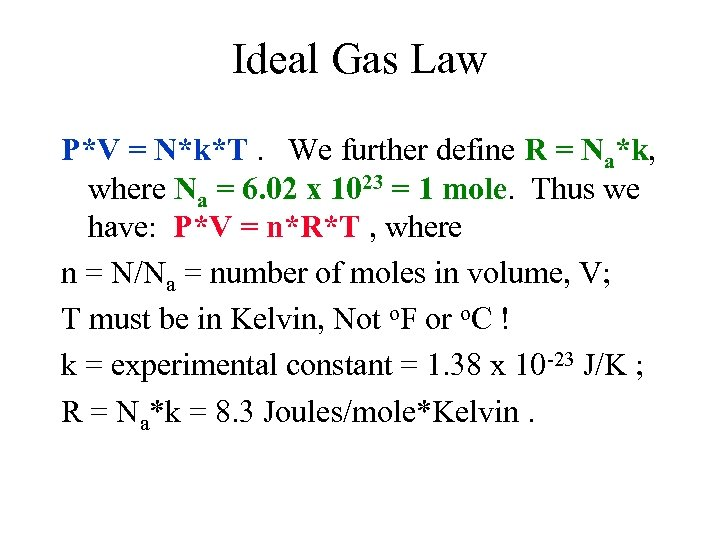Ideal Gas Law P*V = N*k*T. We further define R = Na*k, where Na = 6. 02 x 1023 = 1 mole. Thus we have: P*V = n*R*T , where n = N/Na = number of moles in volume, V; T must be in Kelvin, Not o. F or o. C ! k = experimental constant = 1. 38 x 10 -23 J/K ; R = Na*k = 8. 3 Joules/mole*Kelvin.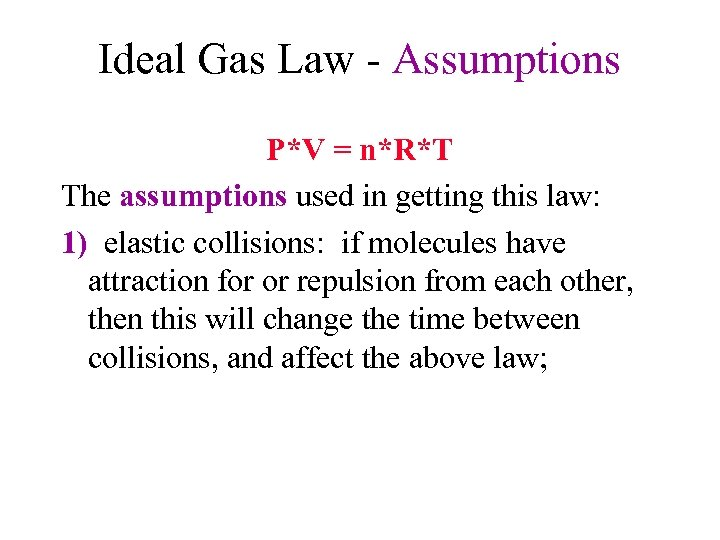Ideal Gas Law - Assumptions P*V = n*R*T The assumptions used in getting this law: 1) elastic collisions: if molecules have attraction for or repulsion from each other, then this will change the time between collisions, and affect the above law;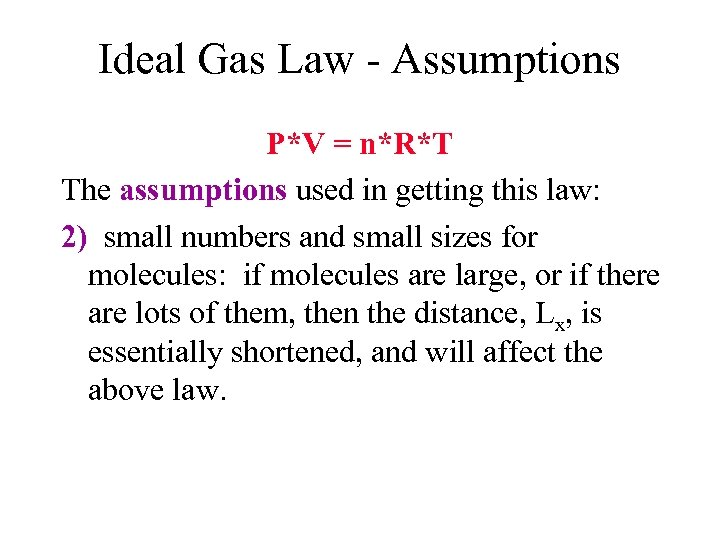Ideal Gas Law - Assumptions P*V = n*R*T The assumptions used in getting this law: 2) small numbers and small sizes for molecules: if molecules are large, or if there are lots of them, then the distance, Lx, is essentially shortened, and will affect the above law.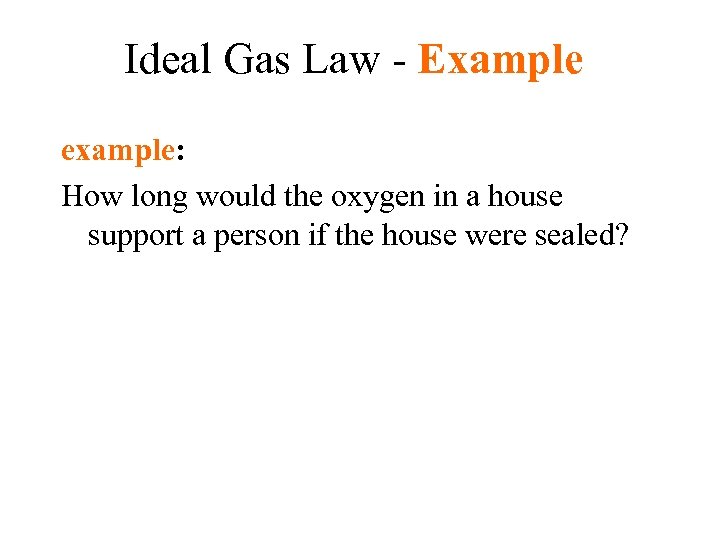Ideal Gas Law - Example example: How long would the oxygen in a house support a person if the house were sealed?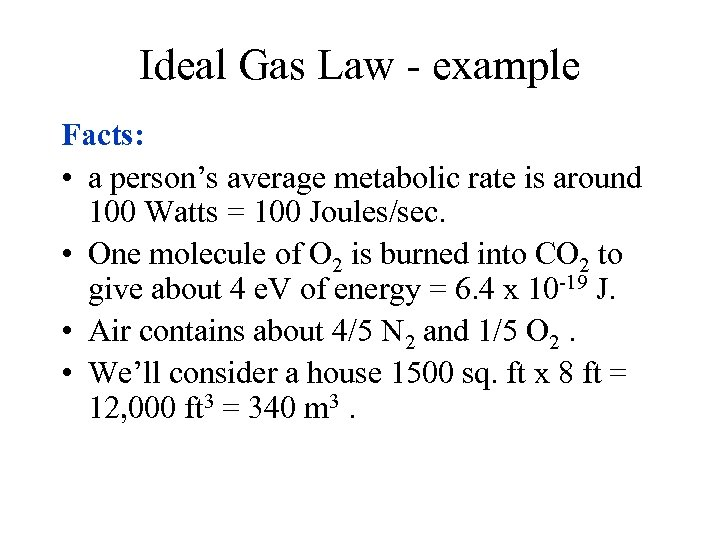Ideal Gas Law - example Facts: • a person’s average metabolic rate is around 100 Watts = 100 Joules/sec. • One molecule of O 2 is burned into CO 2 to give about 4 e. V of energy = 6. 4 x 10 -19 J. • Air contains about 4/5 N 2 and 1/5 O 2. • We’ll consider a house 1500 sq. ft x 8 ft = 12, 000 ft 3 = 340 m 3.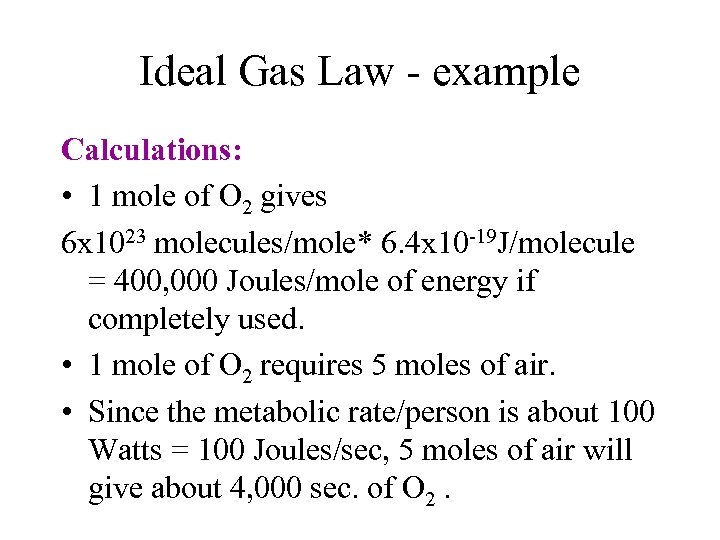Ideal Gas Law - example Calculations: • 1 mole of O 2 gives 6 x 1023 molecules/mole* 6. 4 x 10 -19 J/molecule = 400, 000 Joules/mole of energy if completely used. • 1 mole of O 2 requires 5 moles of air. • Since the metabolic rate/person is about 100 Watts = 100 Joules/sec, 5 moles of air will give about 4, 000 sec. of O 2.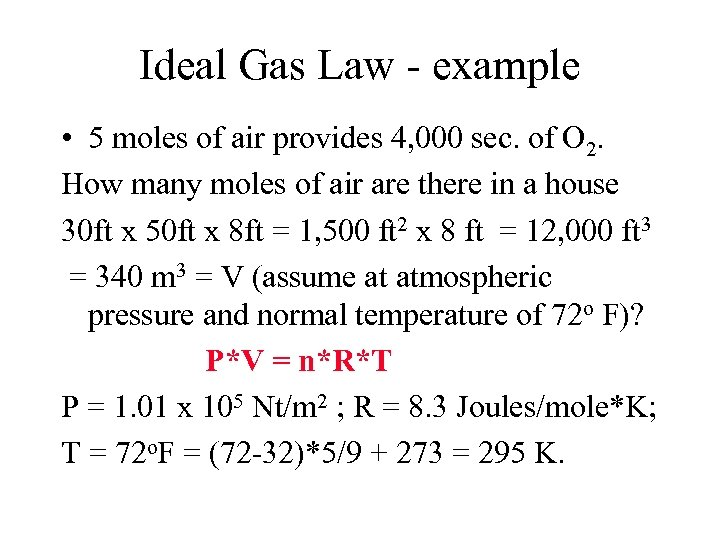Ideal Gas Law - example • 5 moles of air provides 4, 000 sec. of O 2. How many moles of air are there in a house 30 ft x 50 ft x 8 ft = 1, 500 ft 2 x 8 ft = 12, 000 ft 3 = 340 m 3 = V (assume at atmospheric pressure and normal temperature of 72 o F)? P*V = n*R*T P = 1. 01 x 105 Nt/m 2 ; R = 8. 3 Joules/mole*K; T = 72 o. F = (72 -32)*5/9 + 273 = 295 K.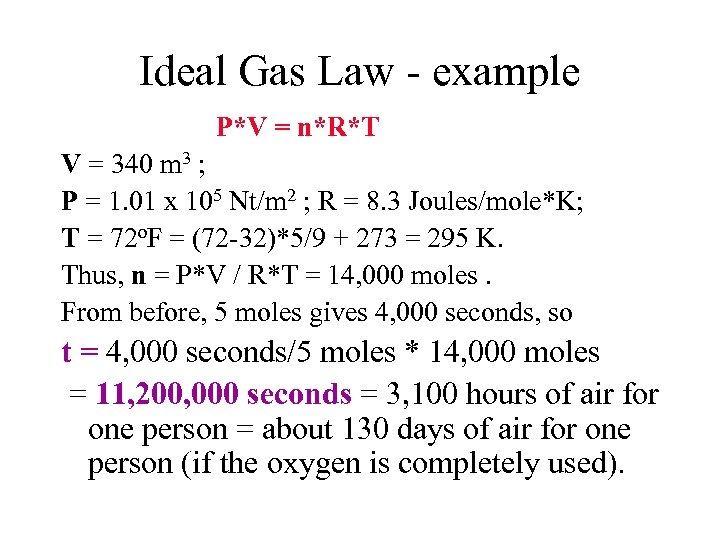Ideal Gas Law - example P*V = n*R*T V = 340 m 3 ; P = 1. 01 x 105 Nt/m 2 ; R = 8. 3 Joules/mole*K; T = 72 o. F = (72 -32)*5/9 + 273 = 295 K. Thus, n = P*V / R*T = 14, 000 moles. From before, 5 moles gives 4, 000 seconds, so t = 4, 000 seconds/5 moles * 14, 000 moles = 11, 200, 000 seconds = 3, 100 hours of air for one person = about 130 days of air for one person (if the oxygen is completely used).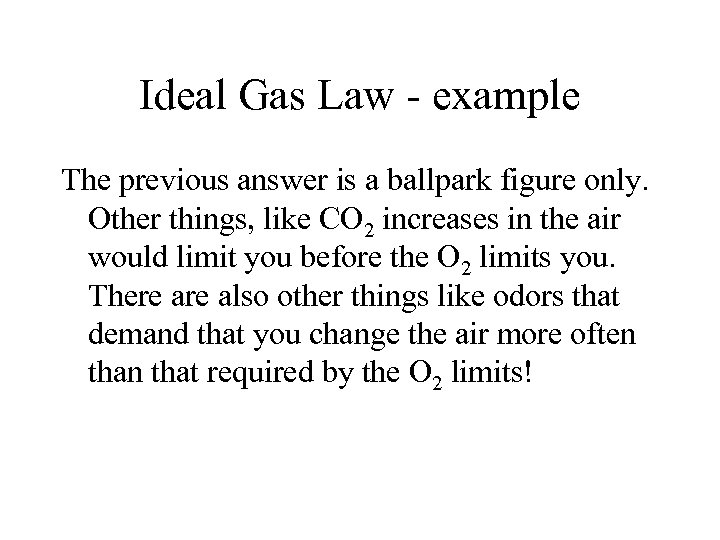Ideal Gas Law - example The previous answer is a ballpark figure only. Other things, like CO 2 increases in the air would limit you before the O 2 limits you. There also other things like odors that demand that you change the air more often that required by the O 2 limits!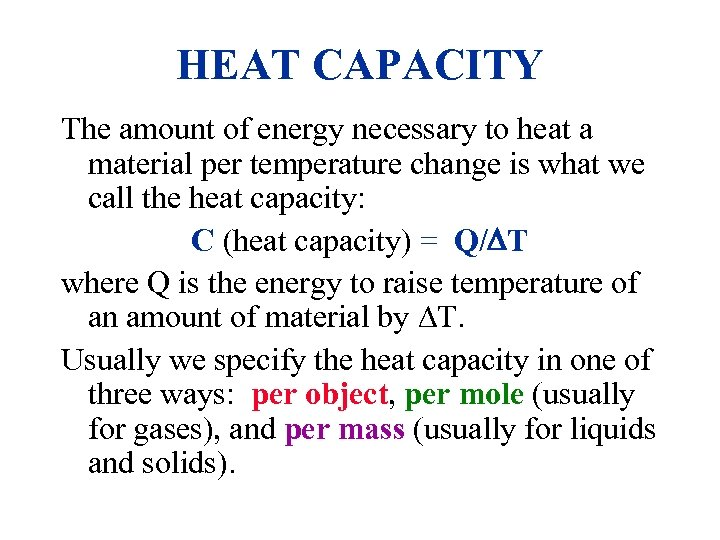HEAT CAPACITY The amount of energy necessary to heat a material per temperature change is what we call the heat capacity: C (heat capacity) = Q/ T where Q is the energy to raise temperature of an amount of material by T. Usually we specify the heat capacity in one of three ways: per object, per mole (usually for gases), and per mass (usually for liquids and solids).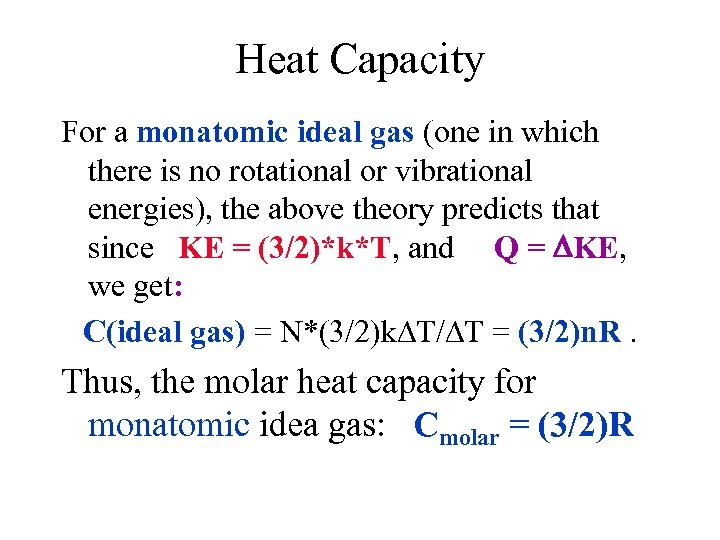Heat Capacity For a monatomic ideal gas (one in which there is no rotational or vibrational energies), the above theory predicts that since KE = (3/2)*k*T, and Q = KE, we get: C(ideal gas) = N*(3/2)k T/ T = (3/2)n. R. Thus, the molar heat capacity for monatomic idea gas: Cmolar = (3/2)R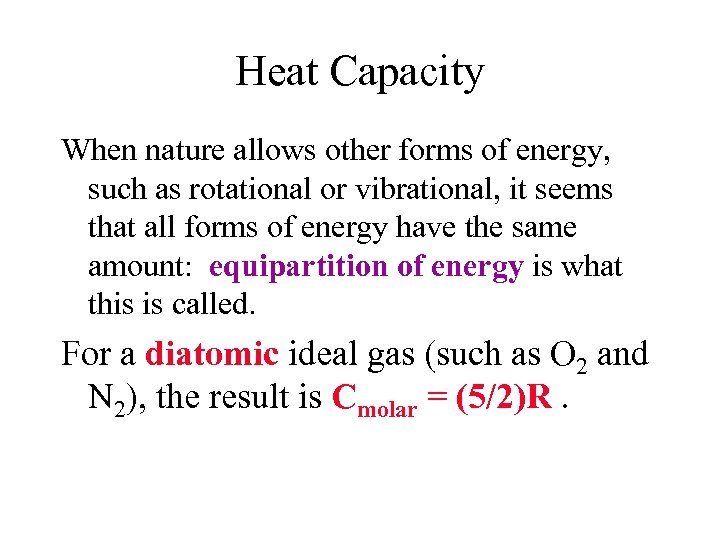Heat Capacity When nature allows other forms of energy, such as rotational or vibrational, it seems that all forms of energy have the same amount: equipartition of energy is what this is called. For a diatomic ideal gas (such as O 2 and N 2), the result is Cmolar = (5/2)R.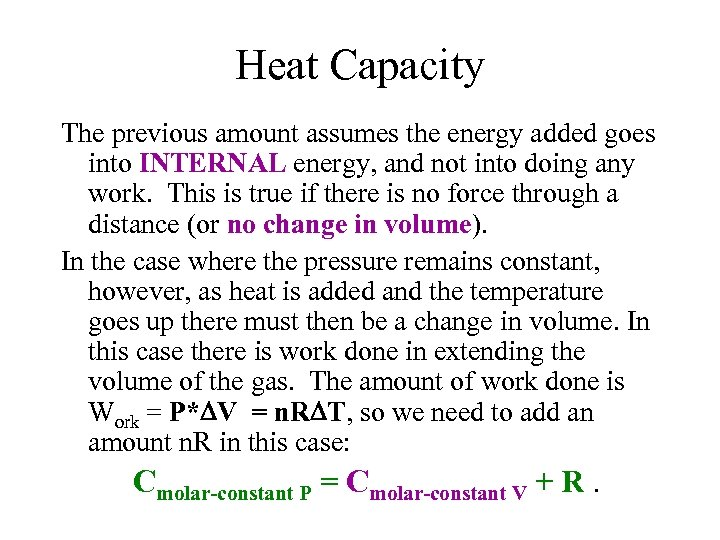Heat Capacity The previous amount assumes the energy added goes into INTERNAL energy, and not into doing any work. This is true if there is no force through a distance (or no change in volume). In the case where the pressure remains constant, however, as heat is added and the temperature goes up there must then be a change in volume. In this case there is work done in extending the volume of the gas. The amount of work done is Work = P* V = n. R T, so we need to add an amount n. R in this case: Cmolar-constant P = Cmolar-constant V + R.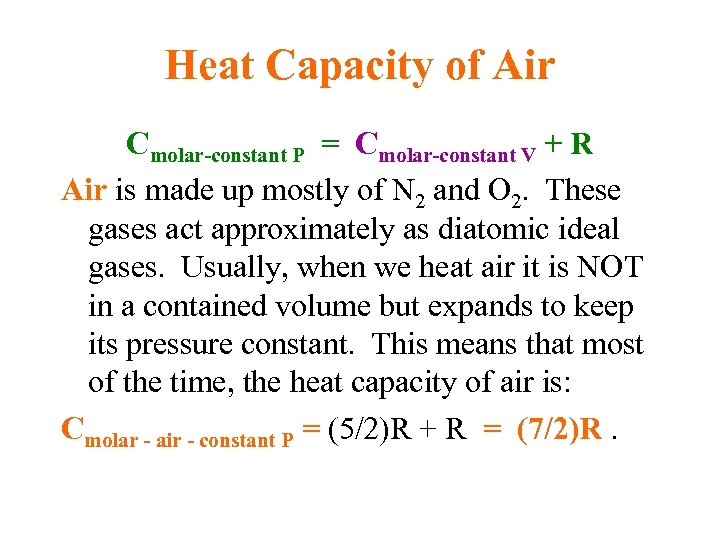Heat Capacity of Air Cmolar-constant P = Cmolar-constant V + R Air is made up mostly of N 2 and O 2. These gases act approximately as diatomic ideal gases. Usually, when we heat air it is NOT in a contained volume but expands to keep its pressure constant. This means that most of the time, the heat capacity of air is: Cmolar - air - constant P = (5/2)R + R = (7/2)R.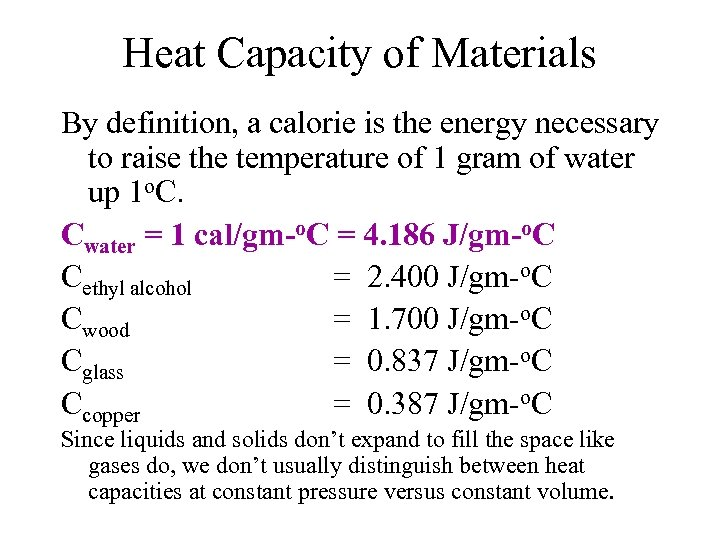Heat Capacity of Materials By definition, a calorie is the energy necessary to raise the temperature of 1 gram of water up 1 o. C. Cwater = 1 cal/gm-o. C = 4. 186 J/gm-o. C Cethyl alcohol = 2. 400 J/gm-o. C Cwood = 1. 700 J/gm-o. C Cglass = 0. 837 J/gm-o. C Ccopper = 0. 387 J/gm-o. C Since liquids and solids don’t expand to fill the space like gases do, we don’t usually distinguish between heat capacities at constant pressure versus constant volume.